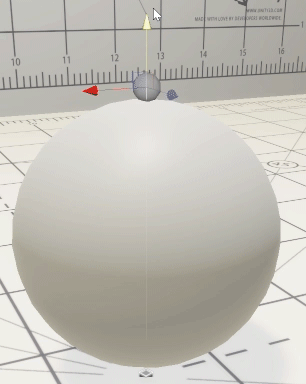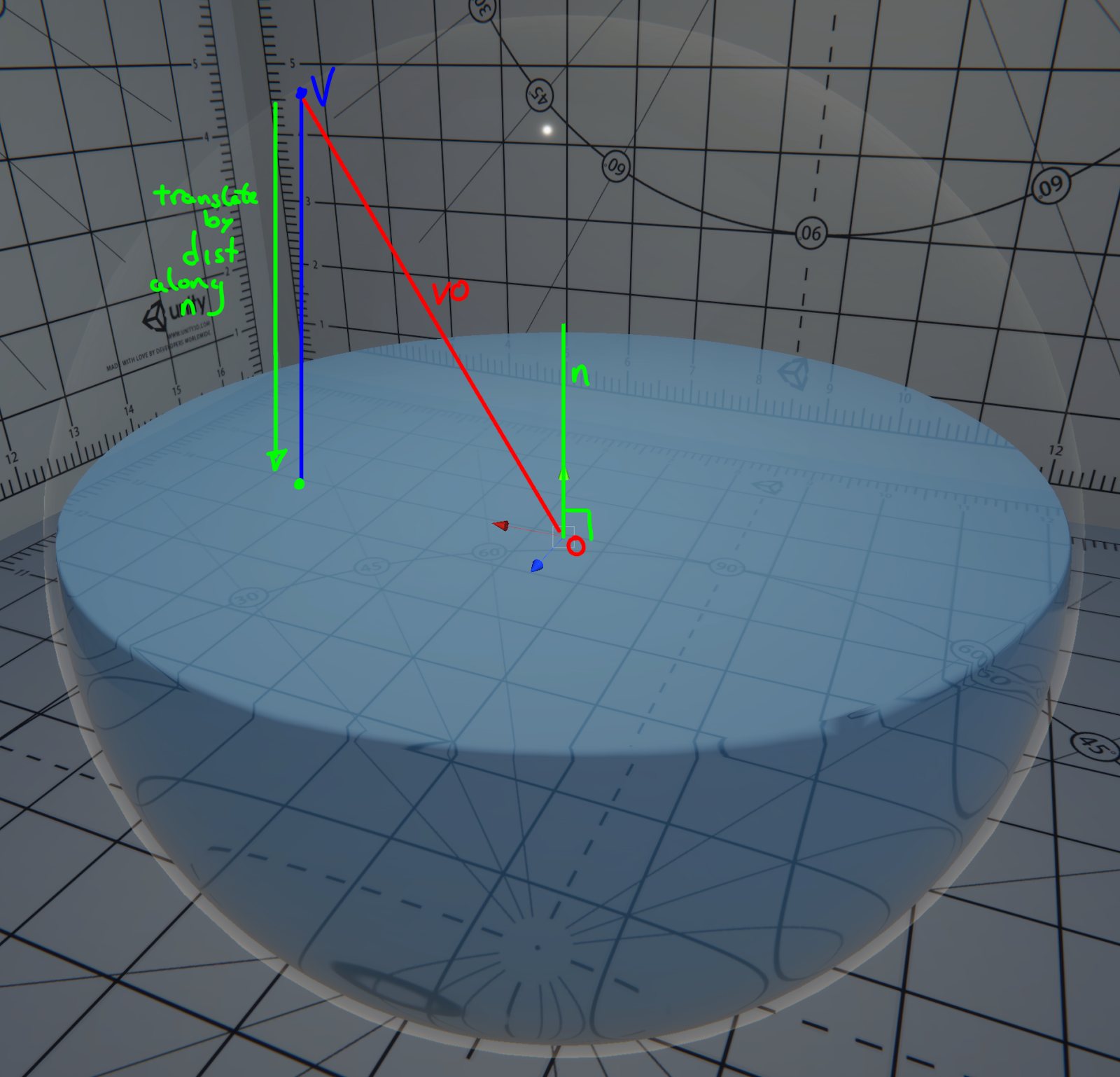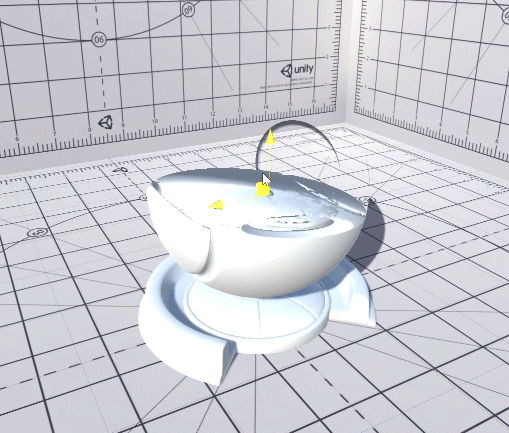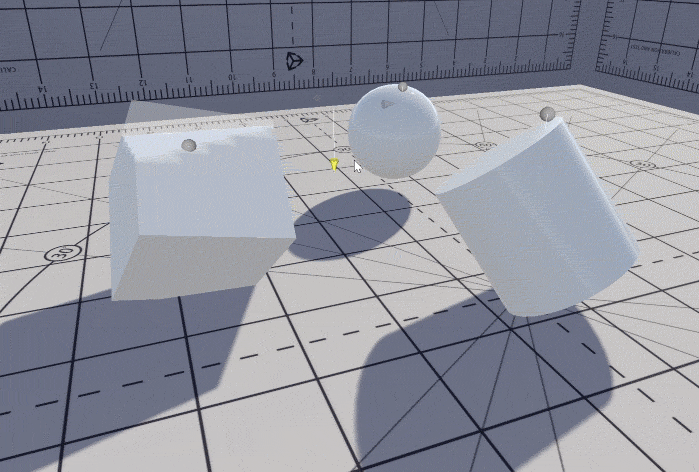# Container Water 01: Flattening the surface

This is the second post (See the first here) in the my “Container Water” series where I’ll be going step by step (more-or-less) into how I’ve approached the creation of this effect for I am Fish. This post goes into detail about where I started with this effect, and at the time of writing the whole system is still heavily WIP.

I chose a continuous surface approach to creating the fish bowl water. This was primarily the breakdown I was following on 80.lv was the clearest example I had at the time, and this was the method Damoiseaux used.

I liked the room this approach provided for adding a 2D fluid sim as we wanted the fish in the bowl to be able to deform the water’s surface.

My rationale behind flattening the fluid was so there’s still a water’s surface to shade for the fluid volume. This is opposed to methods which simply “slice” the volume, leaving a hole in the mesh.

[In hindsight, the surface being continuous doesn’t seem to make this much easier… More on this in a future blog post]

I’d been playing around with some point on plane projection deformation in an older project which never went anywhere, so I quickly got an early water surface example working based on this.

This kind of projection is fairly straightforward;

You take your vertex position, and subtract it from the plane’s origin position.

Then take the dot product of this vector with that unit normal vector of the plane

This is the distance from the plane, to the vertex along the plane’s normal.

I then move the vertex point along the normal by that distance.

Lastly I check to see if that distance is positive.If it is, it means the plane has passed the vertex point and it needs to be flattened, otherwise, we leave it where it is.

And you get something like this.This is what the point on plane projection function looks like. It’s implemented via a custom node in ShaderGraph, as you can see, it’s pretty short.

``````void GlassBowlWater_float(float4 impactPosition,
float4 impactNormal,
float3 vertex,
out float3 Out,
out bool CorrectNormals){
// Get the vector between the plane and the vertex
// Get the dot product between the plane's normal, and the
// planeVertex vector
// projected point is the original point - the dot prod, * the
// plane's normal
float3 vec = impactPosition.xyz - vertex;
float height = dot(impactNormal.xyz, vec);
float3 outVert = height > 0
? vertex + (height * impactNormal.xyz)
: vertex;

Out = height > 0 ? outVert : vertex;
CorrectNormals = height > 0;
}``````

We also output a bool at the end there which we can pass to the rest of our shader if we’ve had to move a vert to become the top of the water’s surface.

This is used to inform other parts of the shaded if they need to recalculate normals.

In the next post in this series, I’ll be looking at constraining the verts to the original volume, to avoid this issue…